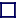### STATISTICAL FACTS

Technetium, Z=43, is the first element in the periodic table for which no stable isotopes exist. The next is promethium, Z=61.

Bismuth, Z=83, is last stable element in the periodic table, all elements above this are radioactive with no stable isotopes.

The number of elements found in nature is 84. The number of stable nuclides is 261, and remarkably, all 261 have been produced in the Universe and moreover are also found on Earth, albeit in differing proportions.

There are no stable isobars of Atomic Weight equal to 5 or 8. Beryllium-8, which might be expected to be stable, lying as it does in the middle of the stability line of the Segre chart, is highly unstable, decaying with a half life of much less than 1/1000th picosecond into two helium-4 nuclei. If beryllium-8 were stable, the fusion reactions within stars would proceed very much more rapidly and explosively. The A=5 and A=8 isobars are the only isobars below A=211 for which there are no stable nuclides.

The first isotone for which no stable isotones exist occurs at N=19. There are many others at N=21, 35, 39, 45, 61, 71, 89, 115 and 123, beyond which most isotones are unstable. Note that these are all odd numbers.